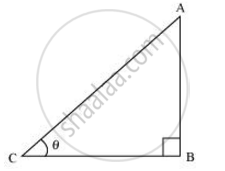# If cot θ = 7/8 evaluate ((1+sin θ )(1-sin θ))/((1+cos θ)(1-cos θ)) - Mathematics

If cot θ = 7/8 evaluate ((1+sin θ )(1-sin θ))/((1+cos θ)(1-cos θ))

#### Solution 1

Let us consider a right triangle ABC, right-angled at point B.cot theta = 7/8

If BC is 7k, then AB will be 8k, where k is a positive integer.

Applying Pythagoras theorem in ΔABC, we obtain

AC2 = AB2 + BC2

= (8k)2 + (7k)2

= 64k2 + 49k2

= 113k2

AC = sqrt113k

sin theta =  (8k)/sqrt(113k) =  8/sqrt(113)

cos theta =  (7k)/sqrt(113k) = 7/sqrt113

((1+sin θ )(1-sin θ))/((1+cos θ)(1-cos θ)) = (1-sin^2θ)/(1-cos^2θ)

= (1-(8/sqrt113)^2)/(1-(7/sqrt(113))^2)= (1-64/113) /(1-49/113)

= (49/113)/(64/113) = 49/64

#### Solution 2

cot theta = 7/8

((1+sin θ )(1-sin θ))/((1+cos θ)(1-cos θ))

= (1 - sin^2 theta)/(1 - cos^2 theta)   [∵ (a + b) (a – b) = a2 − b2] a = 1, b = sin 𝜃

We know that sin 2𝜃 + cos2 𝜃 = 1

1 − sin2 𝜃 = cos2 𝜃 = cos2 𝜃

1 − cos2 𝜃 = sin2 𝜃

= (cos^2 theta)/(sin^2 theta)

= cot^2 theta

= (cot theta)^2 = [7/8]^2

= 49/64

Concept: Trigonometric Ratios
Is there an error in this question or solution?
Chapter 8: Introduction to Trigonometry - Exercise 8.1 [Page 181]

#### APPEARS IN

NCERT Class 10 Maths
Chapter 8 Introduction to Trigonometry
Exercise 8.1 | Q 7.1 | Page 181
RD Sharma Class 10 Maths
Chapter 10 Trigonometric Ratios
Exercise 10.1 | Q 7.1 | Page 24
Share